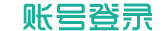# 武汉往事

• [免费]
• [免费]
• [免费]
• [免费]
• [免费]
• [免费]
• [免费]
• [免费]
• [免费]
• [免费]
• [免费]
• [免费]
• [免费]
• [免费]
• [免费]
• [免费]
• [免费]
• [免费]
• [免费]
• [免费]
• [免费]
• [免费]
• [免费]
• [免费]
• [免费]
• [免费]
• [免费]
• [免费]
• [免费]
• [免费]
• [免费]
• [免费]
• [免费]
• [免费]
• [免费]
• [免费]
• [免费]
• [免费]
• [免费]
• [免费]
• [免费]
• [免费]
• [免费]
• [免费]
• [免费]
• [免费]
• [免费]
• [免费]
• [免费]
• [免费]
• [免费]
• [免费]
• [免费]
• [免费]
• [免费]
• [免费]
• [免费]
• [免费]
• [免费]
• [免费]
• [免费]
• [免费]
• [免费]
• [免费]
• [免费]
• [免费]
• [免费]
• [免费]
• [免费]
• [免费]
• [免费]
• [免费]
• [免费]
• [免费]
• [免费]
• [免费]
• [免费]
• [免费]
• [免费]
• [免费]
• [免费]
• [免费]
• [免费]
• [免费]
• [免费]
• [免费]
• [免费]

## 前方进入VIP订阅章节，需要订阅后才能阅读哦！( :